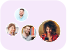Product Edition:1st Edition
Author: Linda J.S. Allen
Book Name: An Introduction to Mathematical Biology
Subject Name: Science

# An Introduction to Mathematical Biology 1st Edition Solutions

0 out of 5.0
56 reviews41 Students
have requested for homework help from this book

For advanced undergraduate and beginning graduate courses on Modeling offered in departments of Mathematics. This text introduces a variety of mathematical models for biological systems, and presents the mathematical theory and techniques useful in analyzing those models. Material is organized according to the mathematical theory rather than the biological application. Undergraduate courses in calculus, linear algebra, and differential equations are assumed.

5
28
4
11
3
15
2
13
1
0

0

## Students who viewed this book also checked out